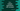# C++ isdigit() function explanation with example## C++ isdigit() function explanation with example:

The isdigit() function is used to check if a given character is decimal digit or not. This function is defined in the cctype header file. In this post, we will learn how to use this function with examples.

### isdigit() function syntax:

The syntax of isdigit function is defined as like below:

``int isdigit(int c)``
• It takes only one argument. It is the character to check. The provided value is casted to an integer or EOF.

#### isdigit() return value:

The isdigit() function returns if the provided value is a decimal digit or not. It returns an integer value. If it is zero, it is not a decimal digit. For decimal digits, it returns a nonzero value.

### Example of isdigit() function:

Let’s try isdigit() function with an example:

``````#include <iostream>
#include <cstring>
#include <cctype>

using namespace std;

int main()
{
char charArr[] = "1abc%\$#-30";

for (int i = 0; i < strlen(charArr); i++)
{
cout << "isdigit(" << charArr[i] << ") = " << isdigit(charArr[i]) << endl;
}
return 0;
}``````

Here,

• charArr is an array of characters, or a string.
• The for loop iterates through the characters of this array one by one and uses isdigit with each of the character.
• Inside the loop, it prints the result of isdigit for the character it is currently iterating.
• The strlen method returns the length of charArr. So, i will start from 0 to length of charArr - 1, i.e. it will iterate through all characters of charArr.
• For strlen, we have to use cstring header and for isdigit, we have to use cctype header.

If you run this program, it will print:

``````isdigit(1) = 1
isdigit(a) = 0
isdigit(b) = 0
isdigit(c) = 0
isdigit(%) = 0
isdigit(\$) = 0
isdigit(#) = 0
isdigit(-) = 0
isdigit(3) = 1
isdigit(0) = 1``````

### Example of isdigit() with user input values:

Let’s use isdigit with user input values. This program will take a character as input from the user and print out if this is a decimal digit or not.

``````#include <iostream>
#include <cctype>

using namespace std;

int main()
{
char c;

cout << "Enter a character: " << endl;
cin >> c;

if (isdigit(c))
{
cout << "It is a decimal digit." << endl;
}
else
{
cout << "It is not a decimal digit." << endl;
}

return 0;
}``````

Here,

• c is a character variable.
• It is asking the user to enter a character. It reads that character and stores that in the variable c.
• The if block is checking if the user entered character is a decimal digit or not.
• If it is a decimal digit, it will enter the if block and print out a message that it is a decimal digit. Else it will enter the else block and print that it is not.

If you run this program, it will print output as like below:

``````Enter a character:
1
It is a decimal digit.

Enter a character:
c
It is not a decimal digit.

Enter a character:
\$
It is not a decimal digit.``````